GET THE APP

Are Thermodynamic Principles Important to Teach in Pharmacy Education?

# Are Thermodynamic Principles Important to Teach in Pharmacy Education?

Antoine Al-Achi*
Campbell University College of Pharmacy & Health Sciences, P.O. Box 1090, Buies Creek, NC 27506, USA
*Corresponding Author: Antoine Al-Achi, Campbell University College of Pharmacy & Health Sciences, P.O. Box 1090, Buies Creek, NC 27506, USA, Tel: (910) 893-1703 x1703, Email: [email protected]

Received Date: Jan 25, 2018 / Accepted Date: Feb 02, 2018 / Published Date: Feb 05, 2018

#### Editorial

Many physical and chemical concepts are highly applicable in pharmacy education. Also, biological principles play a significant role in understanding the fundamentals of drug action and its absorption, distribution, and elimination from the body. Among the physical and chemical information that often taught in pharmacy programs are those related to thermodynamics. In particular, courses in biochemistry, physiology, and pharmaceutics can use thermodynamic concepts to explain the various phenomena in these fields of study. For example, the diffusion of the drug through a biological membrane is a spontaneous process (the word spontaneous implies that the process is natural and irreversible). When drug molecules move from an area of high drug concentration (e.g., the intestine) to an area with low drug concentration (the blood circulation), this pathway is spontaneous. It occurs because all natural systems change in such a way so that further changes are less possible or not possible at all (i.e., they approach a state of equilibrium). These principles can explain the direction of the process, and often they are more applicable nearby an equilibrium point instead of being far away from it. Also, they are more pertinent to isolated closed systems than those encountered in physiological processes. So the dilemma that faces a pharmacy educator is that how to bring these principles into actual practice. It is perhaps best to explain to students about what the various thermodynamic measures mean rather than how to calculate them from observed data. For example, the enthalpy, as a thermodynamic measure, is defined by the amount of heat absorbed or released by a system while the system undergoes a change . This is useful to a student-pharmacist who wants to understand how drug molecules dissolve in a solvent system. During dissolution, the system initially absorbs heat from the environment to break down molecular drug aggregates and in the final step the drug molecules thus released can be deposited in the free cavitation available in the solvent. During that deposition of drug molecules in the cavity of the solvent, the system gives up the heat. 1,2 Another important measure in thermodynamics is the entropy. It is the thermodynamic measure for the amount of energy that is not useful to do work. The higher the entropy of a system, the closer that system to its point of equilibrium and the lower its capacity to do work [1-3]. The pharmacy educator may use for example the states of matter to illustrate the concept of entropy to his students. In this case, a crystalline solid has the lowest entropy, followed by the liquid solute, and then the gaseous/vapor phase of the solute where the entropy value is found at its highest [1,2]. Another illustration for a thermodynamic measure is the concept of free energy. Generally speaking, the free energy of a system is the amount of energy available to a system to perform work. This often is accompanied initially by the system absorbing heat from the environment. Since the free energy is being utilized by the system to do work, it is expected therefore that it decreases in value as the system is doing work. Thus, the difference in free energy must be negative if the system undergoes a spontaneous reaction. If the change in free energy is positive, then no spontaneous response can happen. Another pharmaceutical application of the free energy is in the interfacial phenomenon. The ability of one liquid to spread on the surface of another liquid, when this occurs, an amount of free energy is utilized by the system for the spreading to occur. This energy loss by the system is quantified by the difference between the work of adhesion between the two liquids, and the work of cohesion of the fluid which is spreading onto the surface. By convention, when the work of adhesion is greater in value than the work of cohesion, spreading is possible.

Instructions in this area may also expand to physiological applications where enthalpy, entropy, and free energy can explain whether the process is enthalpy-driven or entropy-driven. Assume that a researcher measures the concentration of potassium ions outside the cell (extracellular fluid) as opposed to that found inside the cell. If the initial concentration of potassium in the extracellular fluid was A1 and that at a final point (i.e., equilibrium or another time point) was A2, then the [2,4] equilibrium constant for this process may be written as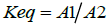(1)

In turn, the equilibrium constant may be calculated at different temperatures, and a linear equation may be found between the natural logarithm of Keq and the inverse of temperature: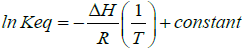(2)

The constant of integration may be estimated from: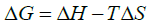(3)

Where, ΔG is the change in free energy, ΔH is the enthalpy, and ΔS is the entropy. The temperature, T, in the equations above must be stated in Kelvin and R is the gas constant.

Also, the change in free energy and Keq are related by the following expression: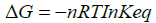(4)

From equations (3) and (4) above and for one mole of the substance (n = 1), we can write,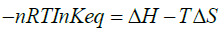(5)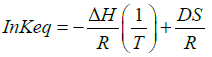(6)

Thus, the constant of integration is obtained from the y-intercept of the straight line between ln Keq vs. (1/T). Therefore, by studying Keq value at different temperatures, the values for ΔH and ΔS will be readily available. Replacing these values in equation (3) above would estimate the change in free energy at a given temperature, T. From equation (3), a higher positive value for S and a higher negative value for H allow the value of ΔG to be more negative, and thus a spontaneous reaction.

The principles of thermodynamics may be introduced in a pharmacy program at many points of the curriculum. Courses such as biochemistry, pharmaceutics, and physiology are all candidates where these concepts may be taught. Emphasis on understanding the measures and how they relate to each other is more important than to show actual calculations for these measures. Perhaps the instructions may be supplemented with scientific articles where students can read how these thermodynamic measures helped in delineating a particular pharmaceutical process (e.g., distinguishing between the various polymorphic forms of a medicinal substance) .

#### References

Citation: Al-Achi A (2018) Are Thermodynamic Principles Important to Teach in Pharmacy Education? Clin Pharmacol Biopharm 7: e130. DOI: 10.4172/2167-065X.1000e130

Copyright: ©2018 Al-Achi A. This is an open-access article distributed under the terms of the Creative Commons Attribution License, which permits unrestricted use, distribution, and reproduction in any medium, provided the original author and source are credited.

Select your language of interest to view the total content in your interested language

Viewmore
##### Open Access Journals
Viewmore
###### Article Usage
• Total views: 4281
• [From(publication date): 0-2018 - Dec 01, 2021]
• Breakdown by view type
• HTML page views: 3952Can't read the image? click here to refresh